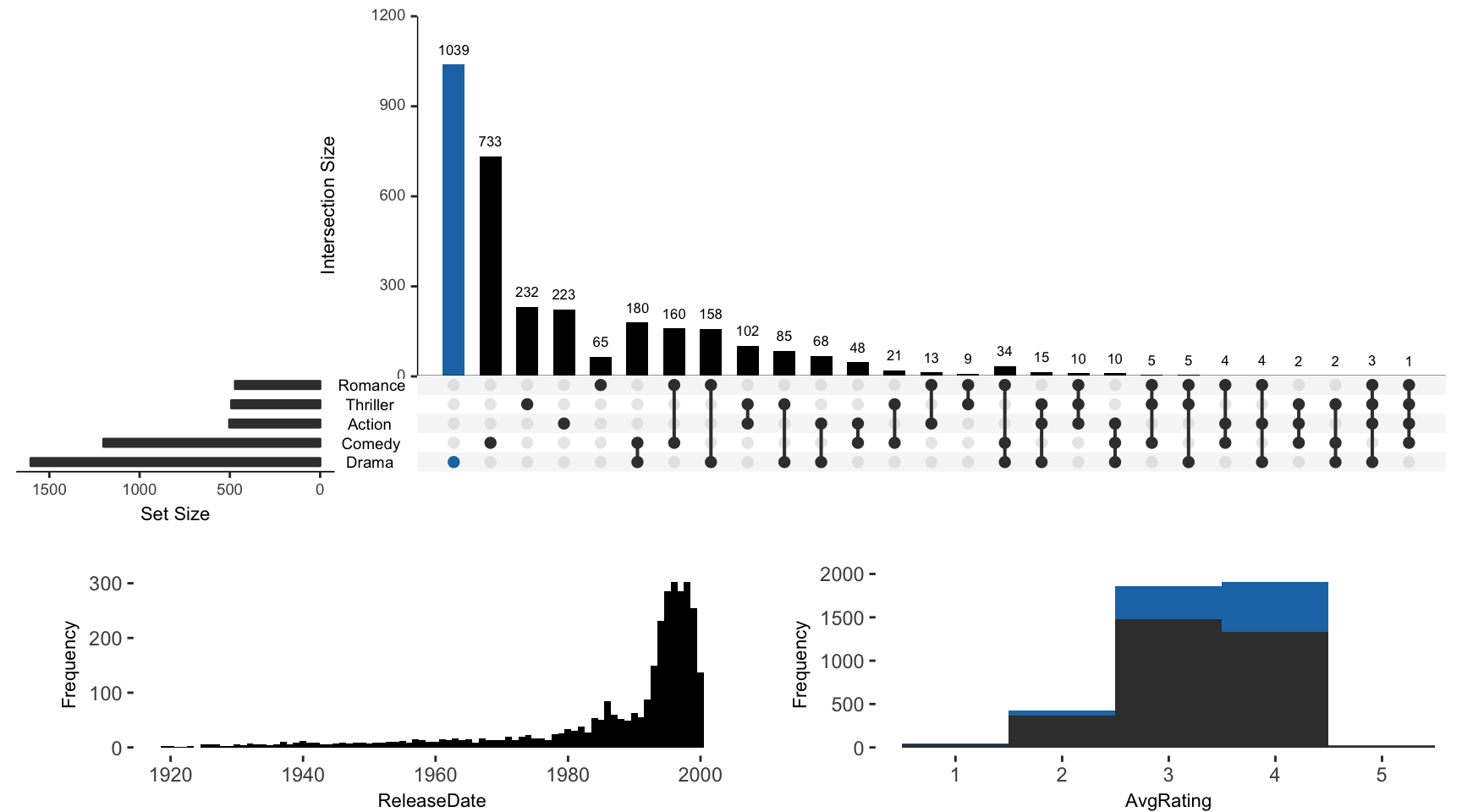For all examples the movies data set contained in the package will be used.

``````library(UpSetR)
library(ggplot2)``````
``## Warning: package 'ggplot2' was built under R version 3.4.4``
``````library(grid)
library(plyr)
movies <- read.csv(system.file("extdata", "movies.csv", package = "UpSetR"),
header = T, sep = ";")``````

## attribute.plots Parameter Breakdown

The `attribute.plots` parameter is broken down into 3 fields: `gridrows`, `plots`, and `ncols`

• `gridrows`: specifies how much to expand the plot window to add room for attribute plots. The UpSetR plot is plotted on a 100 by 100 grid. So for example, if we set `gridrows` to 50, the new grid layout would be 150 by 100, setting aside 1/3 of the plot for the attribute plots.

• `plots`: takes a list of paramters. These paramters include `plot`, `x`, `y` (if applicable), and `queries`.

• `plot`: is a function that returns a ggplot

• `x`: is the x aesthetic to be used in the ggplot (entered as string)

• `y`: is the y aesthetic to be used in the ggplot (entered as string)

• `queries`: indicates whether or not to overlay the plot with the queries present. If `queries` is `TRUE`, the attribute plot will be overlayed with data from the queries. If `queries` is `FALSE`, no query results will be plotted on the attribute plot.

• `ncols`: specifies how the plots should be arranged in the `gridrows` space. If two attribute plots are entered and `ncols` is 1,then the plots will display one above the other. Alternatively, if two attribute plots are entered and `ncols` is 2, the attribute plots will be displayed side by side.

Additional: to add a legend of the queries, use `query.legend = "bottom"` (see Example 2).

## Example 1: Built-In Attribute Histogram

Example of how to add built-in histogram attribute plot. If `main.bar.color` is not specified as black, elements contained in black intersection size bars will be represented as gray in attribute plots.

``````upset(movies, main.bar.color = "black", queries = list(list(query = intersects,
params = list("Drama"), active = T)), attribute.plots = list(gridrows = 50,
plots = list(list(plot = histogram, x = "ReleaseDate", queries = F), list(plot = histogram,
x = "AvgRating", queries = T)), ncols = 2))``````## Example 2: Built-In Attribute Scatter Plot

Example of how to add built-in attribute scatter plot. If `main.bar.color` not specified as black, elements contained in black intersection size bars will be represented as gray in attribute plots.

notice the use of query.legend

``````upset(movies, main.bar.color = "black", queries = list(list(query = intersects,
params = list("Drama"), color = "red", active = F), list(query = intersects,
params = list("Action", "Drama"), active = T), list(query = intersects,
params = list("Drama", "Comedy", "Action"), color = "orange", active = T)),
attribute.plots = list(gridrows = 45, plots = list(list(plot = scatter_plot,
x = "ReleaseDate", y = "AvgRating", queries = T), list(plot = scatter_plot,
x = "AvgRating", y = "Watches", queries = F)), ncols = 2), query.legend = "bottom")``````